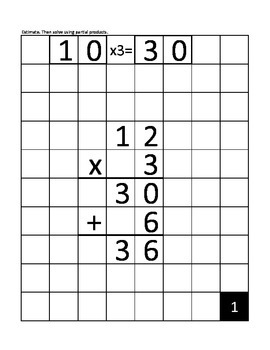# 1-Digit by Up to 4-Digits Multiplication {Math Masters}4th - 6th
Subjects
Standards
Resource Type
Formats Included
• PDF
• Compatible withActivities
Pages
80 pages
Compatible with Easel Activities
This resource is compatible with Easel by TpT, a suite of digital tools you can use to make any lesson interactive and device-ready. Customize this activity and assign it to students, all from Easel. Easel is free to use! Learn more.

### Description

Do your students need to practice solving 1-digit by up to 4-digit multiplication problems? Are you trying to avoid plain, old pencil & paper? Print these problems on card stock & place in a sheet protector for students to solve using a dry erase marker. When done, students simply pull the card stock out of the sheet protector, flip it over, and slide it back in to check their work. They will easily be able to determine whether their answer is correct or not. If not, students will see where they made their mistake to help them learn from it.

Included are 20 math problems. Each problem has two different starting cards - either for partial products or the standard algorithm. Both require students to first estimate the product before they solve it. Answer cards are included for both methods.

Skills Practiced

-Multiply a 1-digit number through the thousands place - including regrouping.

-Estimating

-Multiplying using patterns

CCSS.MATH.CONTENT.4.NBT.B.5

Multiply a whole number of up to four digits by a one-digit whole number, and multiply two two-digit numbers, using strategies based on place value and the properties of operations. Illustrate and explain the calculation by using equations, rectangular arrays, and/or area models.

CCSS.MATH.CONTENT.5.NBT.B.5

Fluently multiply multi-digit whole numbers using the standard algorithm.

-----------------------------------------------------------------------------

Thanks for visiting and checking out my products!

Total Pages
80 pages
Included
Teaching Duration
N/A
Report this Resource to TpT
Reported resources will be reviewed by our team. Report this resource to let us know if this resource violates TpT’s content guidelines.

### Standards

to see state-specific standards (only available in the US).
Fluently multiply multi-digit whole numbers using the standard algorithm.
Multiply a whole number of up to four digits by a one-digit whole number, and multiply two two-digit numbers, using strategies based on place value and the properties of operations. Illustrate and explain the calculation by using equations, rectangular arrays, and/or area models.